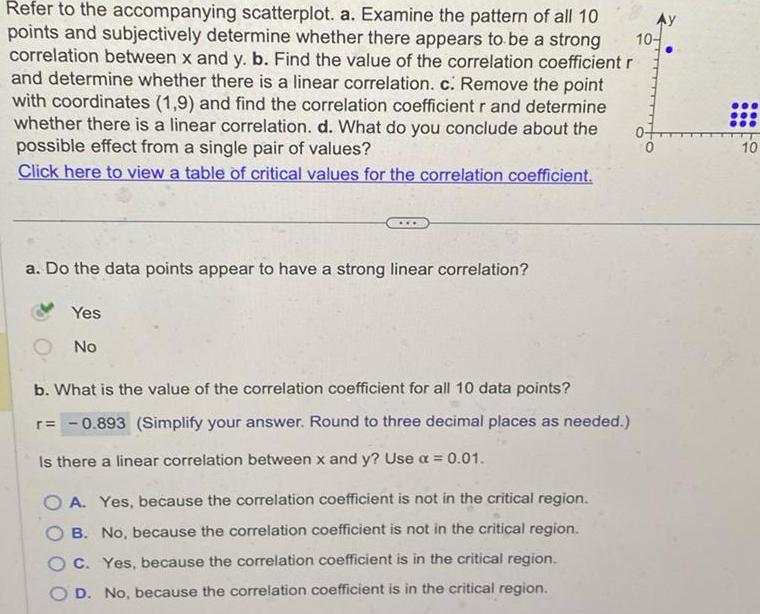Question:

# 10 Refer to the accompanying scatterplot a Examine the

Last updated: 11/20/202310 Refer to the accompanying scatterplot a Examine the pattern of all 10 points and subjectively determine whether there appears to be a strong correlation between x and y b Find the value of the correlation coefficient r and determine whether there is a linear correlation c Remove the point with coordinates 1 9 and find the correlation coefficient r and determine whether there is a linear correlation d What do you conclude about the possible effect from a single pair of values Click here to view a table of critical values for the correlation coefficient a Do the data points appear to have a strong linear correlation Yes No b What is the value of the correlation coefficient for all 10 data points r 0 893 Simplify your answer Round to three decimal places as needed Is there a linear correlation between x and y Use a 0 01 A Yes because the correlation coefficient is not in the critical region B No because the correlation coefficient is not in the critical region C Yes because the correlation coefficient is in the critical region OD No because the correlation coefficient is in the critical region 0 O 10